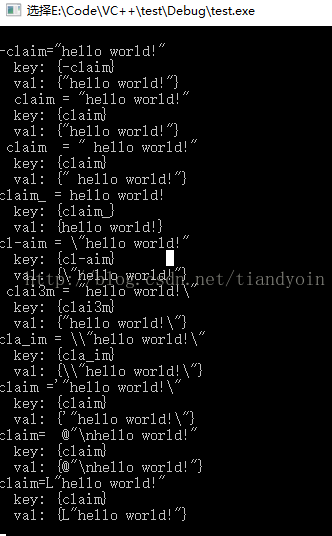# 使用正则表达式(regex_replace)模拟读取INI文件

更新时间：2017年07月12日 15:32:24   作者：tiandyoin我要评论

```#include "stdio.h"
#include <sstream>
#include <iostream>
#include <fstream>
#include <regex>
using namespace std;
void Trim(char * str);
void lTrim(char * str);
void rTrim(char * str);
// 测试sscanf 和 正则表达式
// sscanf提供的这个扩展功能其实并不能真正称为正则表达式，因为他的书写还是离不开%
// []表示字符范围，{}表示重复次数，^表示取非，*表示跳过。所以上面这个url的解析可以写成下面这个样子：
//
//char url[] = "dv://192.168.1.253:65001/1/1"
//
//sscanf(url, "%[^://]%*c%*c%*c%[^:]%*c%d%*c%d%*c%d", protocol, ip, port, chn, type);
//
//解释一下
//先取得一个最长的字符串，但不包括字串 ://，于是protocol="dv\0";
//然后跳过三个字符,（%*c）其实就是跳过 ://
// 接着取一个字符串不包括字符串 : ，于是ip = 192.168.1.253，这里简化处理了，IP就当个字符串来弄，而且不做检查
// 然后跳过冒号取端口到port，再跳过 / 取通道号到chn，再跳过 / 取码流类型到type。
// c语言实现上例
void test1()
{
char url[] = "dv://192.168.1.253:65001/1/1";
char protocol;
char ip;
int port;
int chn;
int type;
sscanf(url, "%[^://]%*c%*c%*c%[^:]%*c%d%*c%d%*c%d", protocol, ip, &port, &chn, &type);
printf("%s, %s, %d, %d, %d\n", protocol, ip, port, chn, type);
}
// 读取ini里某行字符串, 得到: hello world!
// 正常串1： -claim="hello world!"
// 正常串2： claim = "hello world!"
// 正常串3： claim = " hello world!"
// 正常串4： claim_ = hello world!
// 干扰串1： cl-aim = \"hello world!"
// 干扰串2： clai3m = "hello world!\"
// 干扰串3： cla_im = \\"hello world!\"
// 干扰串4： claim ='"hello world!\"
// 干扰串5： claim= @"\nhello world!"
// 干扰串6： claim=L"hello world!"
// 未处理1： claim = 1
// 未处理1： claim = 1
void test2()
{
char line = { 0 };
char val = { 0 };
char key = { 0 };
FILE *fp = fopen("1.txt", "r");
if (NULL == fp)
{
printf("failed to open 1.txt\n");
return ;
}
while (!feof(fp))
{
memset(line, 0, sizeof(line));
fgets(line, sizeof(line) - 1, fp); // 包含了每行的\n
printf("%s", line);
Trim(line);
// 提取等号之前的内容
memset(key, 0, sizeof(key));
// sscanf使用的format不是正则表达式，不能用 \\s 表示各种空白符，即空格或\t,\n,\r,\f
sscanf(line, "%[^ \t\n\r\f=]", key);
//sscanf(line, "%*[^a-zA-Z0-9_-]%[^ \t\n\r\f=]", key);
printf(" key: [%s]\n", key);
// 提取等号之后的内容
memset(val, 0, sizeof(val));
sscanf(line, "%*[^=]%*c%[^\n]", val); // 不包含了每行的换行符
Trim(val);
printf(" val: [%s]\n", val);
// 去除两边双引号
// ...
// 插入map
// map[key]=value;
// string 转 其它类型
// atoi, atol, atof
}
printf("\n");
fclose(fp);
}
// 上例的C++实现
template<class T1, class T2>
inline T1 parseTo(const T2 t)
{
static stringstream sstream;
T1 r;
sstream << t;
sstream >> r;
sstream.clear();
return r;
}
void test3()
{
char val = { 0 };
char key = { 0 };
ifstream fin("1.txt");
string line;
if (fin)
{
while (getline(fin, line)) // line中不包括每行的换行符
{
cout << line << endl;
/// 提取等号之前的内容
// 第1组()表示任意个空格字符，第2组()表示单词(可带_或-),
// 第3组()表示1个以上的空格字符(或=),最后以任意字符串结尾
regex reg("^([\\s]*)([\\w\\-\\_]+)([\\s=]+).*\$");
// 取第2组代替原串
string key = regex_replace(line, reg, "\$2");
cout << " key: {" << key << "}" << endl;
/// 提取等号之后的内容
// 第1组()表示任意个空格字符，第2组()表示单词(可带_或-),
// 第3组()表示1个以上的空格字符(或=),第4组()表示任意个字符，
// 第5组()表示以任意个空格字符（或回车换行符）结尾。
reg = regex("^([\\s]*)([\\w\\-\\_]+)([\\s=]+)(.*)([\\s\\r\\n]*)\$");
// 取第4组代替原串
string val = regex_replace(line, reg, "\$4");
cout << " val: {" << val << "}" << endl;
// 去除两边双引号
// ...
// 插入map
// map[key]=value;
// string 转 其它类型
// int i = parseTo<int>("123");
// float f = parseTo<float>("1.23");
// string str = parseTo<string>(123);
}
}
else // 没有该文件
{
cout << "no such file" << endl;
}
}
void main()
{
//test1();
test2();
test3();
}
void lTrim(char * str)
{
int i, len;
len = strlen(str);
for (i = 0; i<len; i++)
{
if (str[i] != ' ' && str[i] != '\t' && str[i] != '\n' && str[i] != '\r' && str[i] != '\f') break;
}
memmove(str, str + i, len - i + 1);
return;
}
void rTrim(char * str)
{
int i, len;
len = strlen(str);
for (i = len - 1; i >= 0; i--)
{
if ((str[i] != ' ') && (str[i] != 0x0a) && (str[i] != 0x0d) && (str[i] != '\t') && (str[i] != '\f')) break;
}
str[i + 1] = 0;
return;
}
void Trim(char * str)
{
int i, len;
//先去除左边的空格
len = strlen(str);
for (i = 0; i<len; i++)
{
if (str[i] != ' ' && str[i] != '\t' && str[i] != '\n' && str[i] != '\r' && str[i] != '\f') break;
}
memmove(str, str + i, len - i + 1);
//再去除右边的空格
len = strlen(str);
for (i = len - 1; i >= 0; i--)
{
if (str[i] != ' ' && str[i] != '\t' && str[i] != '\n' && str[i] != '\r' && str[i] != '\f') break;
}
str[i + 1] = 0;
return;
}
/*
void Trim(char * str)
{
lTrim(str);
rTrim(str);
}
*/ ```